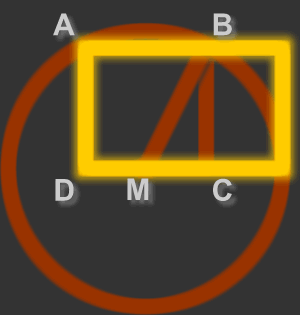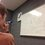# The Golden Ratio: Constructing the Golden RectangleHere is the previous post concerning the Golden Ratio. For a collection of all the posts concerning the Golden Ratio, click #GoldenRatio below.

Today we will learn how to construct the famous Golden Rectangle. Remember that constructions only allow the use of a compass and a straightedge (not a ruler). Simple things that we can do with these devices are make perpendicular lines, find midpoints, draw circles, and bisect angles. It's a fun exercise to follow along with my instructions below.

Here is what I think is the most straightforward way to construct the Golden Rectangle. Take a unit segment. Make a segment perpendicular at the end of the unit segment two units long. Complete the right triangle. We now have a segment measuring $\sqrt{5}$ units. Take this segment and extend it one unit. Then draw the segment that is the average of these two (to do this, find the midpoint of that segment). Then we have a segment measuring $\phi$ units. We can then easily construct a rectangle with dimensions $\phi$ and $1$.

There is, however, a more elegant way. Reference the picture above. Begin by constructing square $ABCD$. Then, construct midpoint $M$ of $CD$. Draw the circle with radius $MB$. Extend $DC$ until it intersects the circle. Call this point of intersection $X$. Finish the rectangle with vertices $ADX$. This rectangle is a Golden Rectangle. Note also that you have created another Golden Rectangle in the process.

Can you prove that this second construction actually produces a golden rectangle? How is this similar to my construction? Post your answers or inquiries below.

The Next PostNote by Bob Krueger
6 years, 10 months ago

This discussion board is a place to discuss our Daily Challenges and the math and science related to those challenges. Explanations are more than just a solution — they should explain the steps and thinking strategies that you used to obtain the solution. Comments should further the discussion of math and science.

When posting on Brilliant:

• Use the emojis to react to an explanation, whether you're congratulating a job well done , or just really confused .
• Ask specific questions about the challenge or the steps in somebody's explanation. Well-posed questions can add a lot to the discussion, but posting "I don't understand!" doesn't help anyone.
• Try to contribute something new to the discussion, whether it is an extension, generalization or other idea related to the challenge.

MarkdownAppears as
*italics* or _italics_ italics
**bold** or __bold__ bold
- bulleted- list
• bulleted
• list
1. numbered2. list
1. numbered
2. list
Note: you must add a full line of space before and after lists for them to show up correctly
paragraph 1paragraph 2

paragraph 1

paragraph 2

[example link](https://brilliant.org)example link
> This is a quote
This is a quote
    # I indented these lines
# 4 spaces, and now they show
# up as a code block.

print "hello world"
# I indented these lines
# 4 spaces, and now they show
# up as a code block.

print "hello world"
MathAppears as
Remember to wrap math in $$ ... $$ or $ ... $ to ensure proper formatting.
2 \times 3 $2 \times 3$
2^{34} $2^{34}$
a_{i-1} $a_{i-1}$
\frac{2}{3} $\frac{2}{3}$
\sqrt{2} $\sqrt{2}$
\sum_{i=1}^3 $\sum_{i=1}^3$
\sin \theta $\sin \theta$
\boxed{123} $\boxed{123}$

Sort by:

since triangleBMC is congruent AND EQUAL to triangle BCX,the new rectangle thus formed is also a goldenrectangle,which is of unit lengthg

- 6 years, 10 months ago